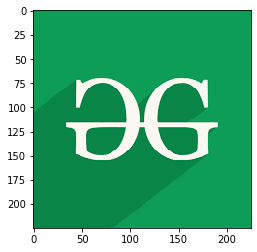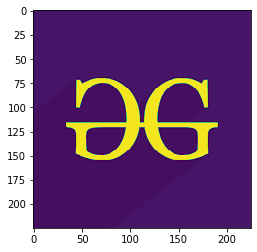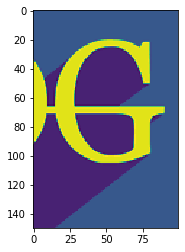Open In App
Related Articles
• Write an Interview Experience

# Working with Images in Python using Matplotlib

Matplotlib is an amazing visualization library in Python for 2D plots of arrays. Matplotlib is a multi-platform data visualization library built on NumPy arrays and designed to work with the broader SciPy stack.

## Working with Images in Python using Matplotlib

The `image` module in `matplotlib` library is used for working with images in Python. The `image` module also includes two useful methods which are `imread` which is used to read images and `imshow` which is used to display the image.

Below are some examples which illustrate various operations on images using `matplotlib` library:

Example 1: In this example, the program reads an image using the `matplotlib.image.imread()` and displays that image using `matplotlib.image.imread()`.

 `# importing required libraries``import` `matplotlib.pyplot as plt``import` `matplotlib.image as img`` ` `# reading the image``testImage ``=` `img.imread(``'g4g.png'``)`` ` `# displaying the image``plt.imshow(testImage)`

Output:Example 2: The below program reads an image and then represents the image in array.

 `# importing required libraries``import` `matplotlib.pyplot as plt``import` `matplotlib.image as img`` ` `# reading the image``testImage ``=` `img.imread(``'g4g.png'``)`` ` `# displaying the image as an array``print``(testImage)`

Output:

```[[[0.05490196 0.6156863  0.34117648]
[0.05490196 0.6156863  0.34117648]
[0.05490196 0.6156863  0.34117648]
...
[0.05490196 0.6156863  0.34117648]
[0.05490196 0.6156863  0.34117648]
[0.05490196 0.6156863  0.34117648]]

[[0.05490196 0.6156863  0.34117648]
[0.05490196 0.6156863  0.34117648]
[0.05490196 0.6156863  0.34117648]
...
[0.05490196 0.6156863  0.34117648]
[0.05490196 0.6156863  0.34117648]
[0.05490196 0.6156863  0.34117648]]

[[0.05490196 0.6156863  0.34117648]
[0.05490196 0.6156863  0.34117648]
[0.05490196 0.6156863  0.34117648]
...
[0.05490196 0.6156863  0.34117648]
[0.05490196 0.6156863  0.34117648]
[0.05490196 0.6156863  0.34117648]]

...

[[0.03529412 0.52156866 0.28235295]
[0.03529412 0.52156866 0.28235295]
[0.03529412 0.52156866 0.28235295]
...
[0.05490196 0.6156863  0.34117648]
[0.05490196 0.6156863  0.34117648]
[0.05490196 0.6156863  0.34117648]]

[[0.03529412 0.52156866 0.28235295]
[0.03529412 0.52156866 0.28235295]
[0.03529412 0.52156866 0.28235295]
...
[0.05490196 0.6156863  0.34117648]
[0.05490196 0.6156863  0.34117648]
[0.05490196 0.6156863  0.34117648]]

[[0.03529412 0.52156866 0.28235295]
[0.03529412 0.52156866 0.28235295]
[0.03529412 0.52156866 0.28235295]
...
[0.05490196 0.6156863  0.34117648]
[0.05490196 0.6156863  0.34117648]
[0.05490196 0.6156863  0.34117648]]]
```

Example 3: Here, the shape of the image is `(225, 225, 3)` which represents (height, width, mode) of the image, for colored image mode value is from 0 to 2 and for black and white image mode value is 0 and 1 only. In the output image, only the mode of the image is modified.

 `# importing required libraries``import` `matplotlib.pyplot as plt``import` `matplotlib.image as img`` ` `# reading the image``testImage ``=` `img.imread(``'g4g.png'``)`` ` `# displaying the shape of the image``print``(testImage.shape)`` ` `# modifying the shape of the image``modifiedImage ``=` `testImage[:, :, ``0``]`` ` `# displaying the modified image``plt.imshow(modifiedImage)`

Output:

`(225, 225, 3)`Example 4: In the below program, all the parameters of the shape of the image are modified. Here the height of the image is 150 pixels (displaying from the 50th pixel), width is 100 pixels (displaying from the 100th pixel) and mode value is 1.

 `# importing required libraries``import` `matplotlib.pyplot as plt``import` `matplotlib.image as img`` ` `# reading the image``testImage ``=` `img.imread(``'g4g.png'``)`` ` `# displaying the shape of the image``print``(testImage.shape)`` ` `# modifying the shape of the image``modifiedImage ``=` `testImage[``50``:``200``, ``100``:``200``, ``1``]`` ` `# displaying the modified image``plt.imshow(modifiedImage)`

Output:

`(225, 225, 3)`Example 5: Here, none of the parameters are modified. So, the original image is displayed.

 `# importing required libraries``import` `matplotlib.pyplot as plt``import` `matplotlib.image as img`` ` `# reading the image``testImage ``=` `img.imread(``'g4g.png'``)`` ` `# displaying the shape of the image``print``(testImage.shape)`` ` `# modifying the shape of the image``modifiedImage ``=` `testImage[:, :, :]`` ` `# displaying the modified image``plt.imshow(modifiedImage)`

Output:

`(225, 225, 3)`